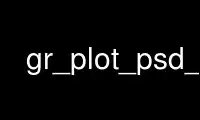# gr_plot_psd_c - Online in the CloudThis is the command gr_plot_psd_c that can be run in the OnWorks free hosting provider using one of our multiple free online workstations such as Ubuntu Online, Fedora Online, Windows online emulator or MAC OS online emulator

### PROGRAM:

#### NAME

gr_plot_psd_c - plot power spectral density using GNU Radio

#### SYNOPSIS

gr_plot_psd_c: [options] input_filename

#### DESCRIPTION

Takes a GNU Radio binary file (with specified data type using --data-type) and displays
the I&Q data versus time as well as the power spectral density (PSD) plot. The y-axis
values are plotted assuming volts as the amplitude of the I&Q streams and converted into
dBm in the frequency domain (the 1/N power adjustment out of the FFT is performed
internally). The script plots a certain block of data at a time, specified on the command
line as -B or --block. The start position in the file can be set by specifying -s or
--start and defaults to 0 (the start of the file). By default, the system assumes a sample
rate of 1, so in time, each sample is plotted versus the sample number. To set a true time
and frequency axis, set the sample rate (-R or --sample-rate) to the sample rate used when
capturing the samples. Finally, the size of the FFT to use for the PSD and spectrogram
plots can be set independently with --psd-size and --spec-size, respectively. The
spectrogram plot does not display by default and is turned on with -S or --enable-spec.

#### OPTIONS

-h, --help
show this help message and exit

-B BLOCK, --block=BLOCK
Specify the block size [default=8192]

-s START, --start=START
Specify where to start in the file [default=0]

-R SAMPLE_RATE, --sample-rate=SAMPLE_RATE
Set the sampler rate of the data [default=1.0]

--psd-size=PSD_SIZE
Set the size of the PSD FFT [default=1024]

--spec-size=SPEC_SIZE
Set the size of the spectrogram FFT [default=256]

-S, --enable-spec
Turn on plotting the spectrogram [default=False]

Use gr_plot_psd_c online using onworks.net services

Free Servers & Workstations